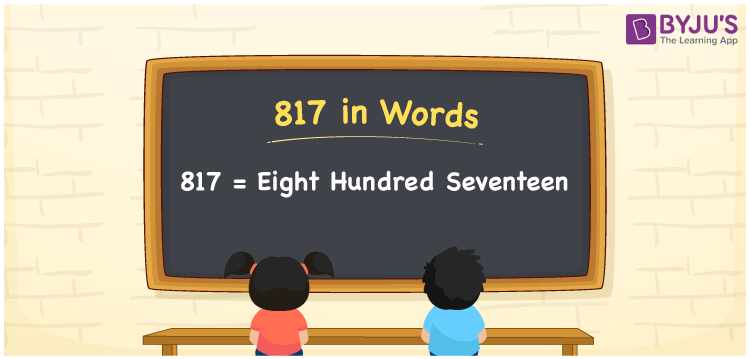# 817 in Words

817 in words is written as Eight hundred seventeen. In both the International System of Numerals and the Indian System of Numerals, 817 is written as Eight hundred seventeen. The number 817 is a Cardinal Number as it represents some quantity. For example, “817 dishes are required”.

 817 in Words Eight hundred seventeen Eight hundred seventeen in Number 817

## 817 in English Words

We write 817 in English Words using the letters of the English alphabet. Therefore, we read 817 in English as “Eight hundred seventeen.”## How to Write 817 in Words?

To write 817 in words, we shall use the place value chart. In the place value chart, write 8 in the hundreds, 1 in the tens, and 7 in the ones, respectively. Now let us make a place value chart to write the number 817 in words.

 Hundreds Tens Ones 8 1 7

Thus, we can write the expanded form as

8 × Hundred + 1 × Ten + 7 × One

= 8 × 100 + 1 × 10 + 7 × 1

= 800 + 10 + 7

= 817

= Eight hundred seventeen.

817 is a natural number, the successor of 816 and the predecessor of 818.

817 in words – Eight hundred seventeen

• Is 817 an odd number? – Yes
• Is 817 an even number? – No
• Is 817 a perfect square number? – No
• Is 817 a perfect cube number? – No
• Is 817 a prime number? – No
• Is 817 a composite number? – Yes

## Frequently Asked Questions on 817 in Words

Q1

### How to write 817 in words?

817 in words is written as Eight hundred seventeen.
Q2

### How to write 817 in the International and Indian System of Numerals?

In both, the system of numerals, 817 in words, is written as Eight hundred seventeen.
Q3

### What is the number that comes after 817?

The number that comes after 817 is 818.